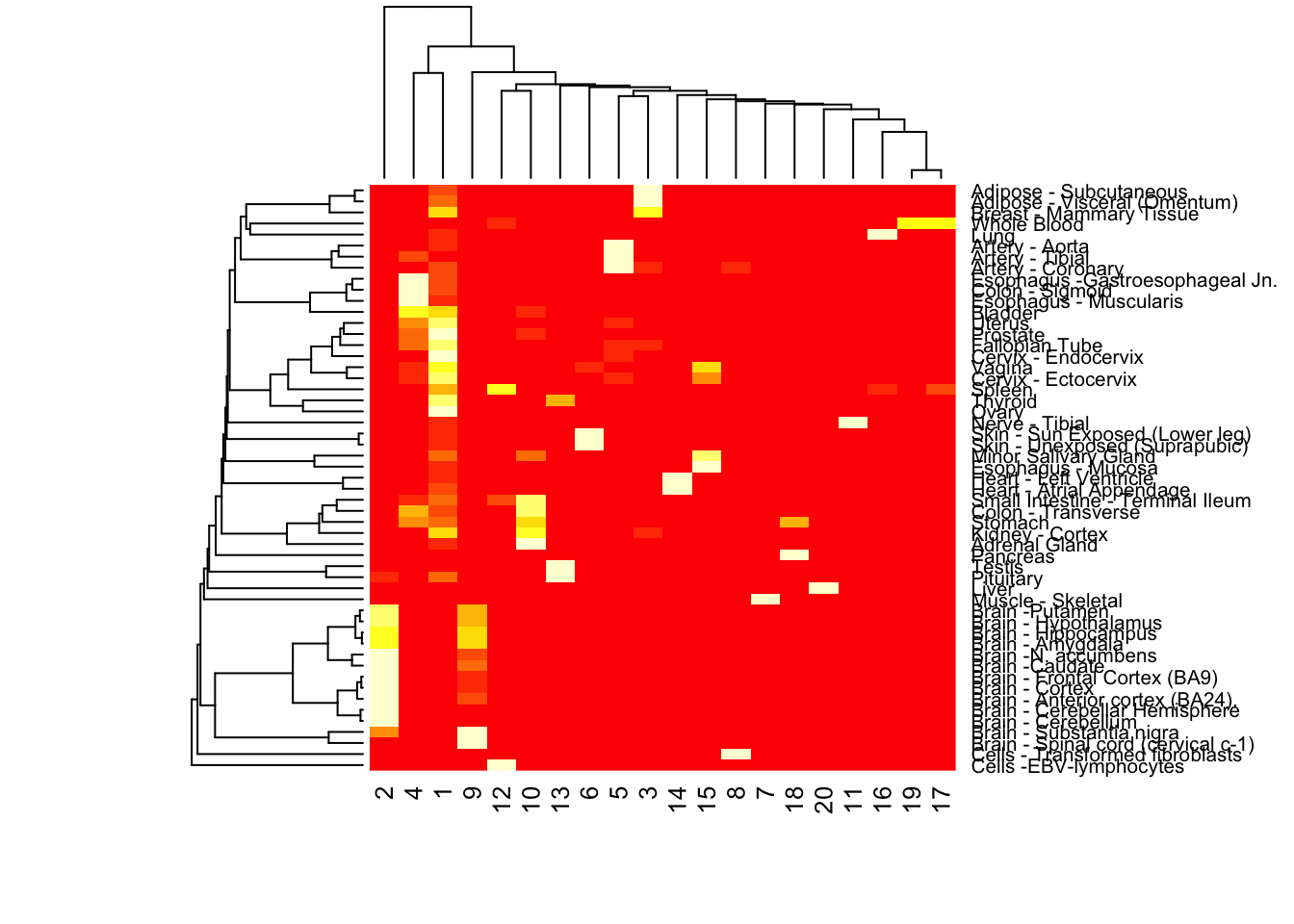## Objective

In this script, we perform the topic model and Structure plots on the GTEX V6 data across all tissues and then exclusively on the brain tissues. We fit a topic model with \(K=15\) topics over all tissues in the GTEX study and then plot the Structure plot. We also fit a topic model with \(4\) topics on the brain data exclusively and then plot the corresponding Structure plot as well.

``````rm(list=ls())
library(data.table)
#install_github('kkdey/maptpx')
library(maptpx)
library(CountClust)
library(data.table)``````

## Data preprocessing

``data <- data.frame(fread('../external_data/GTEX_V6/cis_gene_expression.txt'));``
``````##
Read 0.0% of 16069 rows
Read 62.2% of 16069 rows
Read 16069 rows and 8557 (of 8557) columns from 0.532 GB file in 00:00:09``````
``````matdata <- data[,-(1:2)];
samples_id=read.table("../external_data/GTEX_V6/samples_id.txt")[,3];``````

We fit a topic model for \(K=20\) over all the tissues in the GTEX V6 data.

``````Topic_Clus=topics(t(matdata),15,kill=0,tol=0.1);
docweights=Topic_Clus\$omega;``````

## Structure Plot (All tissues)

``````gom_model_fit <- get(load("../external_data/GTEX_V6/gtexv6fit.k.20.master.rda"))
omega <- gom_model_fit\$omega
colnames(omega) <- c(1:NCOL(omega))

# make cell sample labels
# want a version consistent with majority of the literature
sample_labels <- read.table("../external_data/GTEX_V6/samples_id.txt",
header = TRUE, sep = " ",
stringsAsFactors = FALSE)
tissue_labels <- vector("numeric", NROW(sample_labels))
tissue_labels <- sample_labels[ ,3]

# clean labels
tissue_labels[grep("Nucleus", tissue_labels)] <- "Brain -N. accumbens"
tissue_labels[grep("Putamen", tissue_labels)] <- "Brain -Putamen"
tissue_labels[grep("Caudate", tissue_labels)] <- "Brain -Caudate"
tissue_labels[grep("Gastroe", tissue_labels)] <- "Esophagus -Gastroesophageal Jn."
tissue_labels[grep("cingulate", tissue_labels)] <- "Brain - Anterior cortex (BA24)."
tissue_labels[grep("EBV", tissue_labels)] <- "Cells -EBV-lymphocytes"
tissue_labels[grep("Suprapubic", tissue_labels)] <- "Skin - Unexposed (Suprapubic)"
tissue_labels[grep("Lower Leg", tissue_labels)] <- "Skin - Sun Exposed (Lower Leg)"

# find sample orders in hierarchical clustering
docweights_per_tissue_mean <- apply(omega, 2,
function(x) { tapply(x, tissue_labels, mean) })
ordering <- heatmap(docweights_per_tissue_mean)\$rowInd````````````# order tissue by hierarhical clustering results
tissue_levels_reordered <- unique(tissue_labels)[ordering]

annotation <- data.frame(
sample_id = paste0("X", 1:length(tissue_labels)),
tissue_label = factor(tissue_labels,
levels = rev(tissue_levels_reordered ) ) )

cols1 <- c(rev(RColorBrewer::brewer.pal(12, "Paired"))[c(3,4,7,8,11,12,5,6,9,10)],
RColorBrewer::brewer.pal(12, "Set3"))``````
``````StructureGGplot(omega = omega,
annotation= annotation,
palette = cols1,
yaxis_label = "",
order_sample = TRUE,
split_line = list(split_lwd = .1,
split_col = "white"),
axis_tick = list(axis_ticks_length = .1,
axis_ticks_lwd_y = .1,
axis_ticks_lwd_x = .1,
axis_label_size = 5,
axis_label_face="bold"))``````